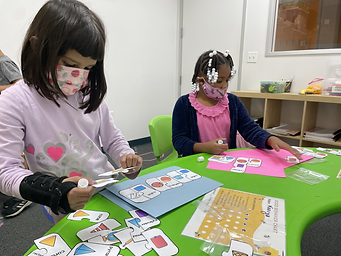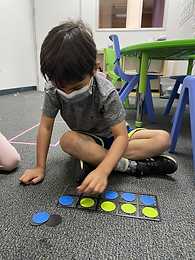## Ms. Caitlyn

### Target 1​

###### Lesson Type:

Continuation

Geometry

:

Shape

Identify and compare 2D geometric shapes based on their attributes, regardless of orientation or size.

###### 1:

Identify basic 3D shapes and their defining attributes (cone, sphere, cube, and cylinder).

###### 2:

Identify the number of faces, number of edges, number of corners (vertices), and measurements of 3D shapes.

###### 3:

Identify basic geometric shapes that best represent real-world objects.

1st

###### Vocabulary:

Face, Edge, Vertices

Activities:

Students counted faces, edges, and vertices on 3D foam blocks.

Students created cubes and rectangular prisms with Rising Stars.

Students completed puzzles where they matched 3D figures (cone, sphere, cylinder, pyramid, rectangular prism, cube) to real-world objects.### Home Exploration

###### Guiding Questions:## Absent Students:

### Target 2

:

###### 1:

Compose larger shapes from smaller shapes (two small triangles can make a rectangle).

###### 2:

Study geometric shapes to derive the component shapes that can be used to create it.

1st

###### Vocabulary:

Activities:

Students used different shapes to create larger shapes (ex.: six small triangles make one hexagon).### Home Exploration

###### Guiding Questions:### Target 3

:

###### 1:

Identify the correct arithmetic process based on the information presented in word problems.

###### 2:

Find the key words in word problems that indicate the correct arithmetic process to use (all together is addition, take away is subtraction, etc.).

###### 3:

Find and use the needed information in a word problem in order to solve.

1st

###### Vocabulary:

Activities:

Students listened to the teacher read word problems out loud and identified key words, indicating whether to add or to subtract.

Students used ten frames to model problems within 10.

Students used blocks to model problems within 40.

Students turned word problems into number sentences by identifying which numbers and operations to use.### Home Exploration